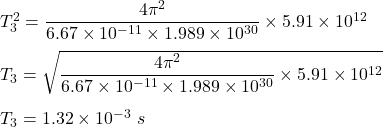## The orbital radius of the Earth (from Earth to Sun) is 1.496 x 10^11 m. Mercury’s orbital radius is 5.79 x 10^10 m and Pluto’s is 5.91

Question

The orbital radius of the Earth (from Earth to Sun) is 1.496 x 10^11 m.
Mercury’s orbital radius is 5.79 x 10^10 m and Pluto’s is 5.91 x 10^12 m.
Calculate the time required for light to travel from the Sun to each of the
three planets.
a. Sun-Earth:_______
b. Sun-Mercury:________
c. Sun-Pluto:________​

in progress 0
4 months 2021-09-05T15:10:33+00:00 1 Answers 6 views 0

1. Explanation:

The orbital radius of the Earth is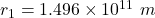The orbital radius of the Mercury is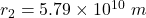The orbital radius of the Pluto is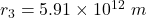We need to find the time required for light to travel from the Sun to each of the  three planets.

(a) For Sun -Earth,

Kepler’s third law :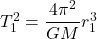M is mass of sun,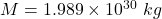So,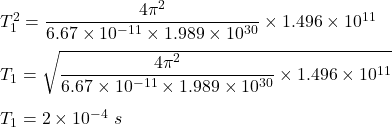(b) For Sun -Mercury,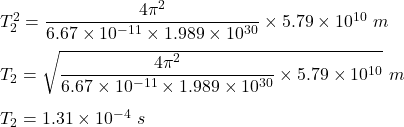(c) For Sun-Pluto,